# Variance: Order out of Chaos

In the previous post, I wrote about expectation, a great tool that helps us capture notion of “center” for a random variable. In this post, I will write about variance, another great tool that represents the “spread” of a random variable.

Consider a random variable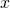with two probability density functions (PDFs)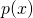and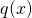, shown in the figure below.under both PDFs has the same expected value (i.e.,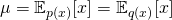). But,has a larger “spread” compared to. In other words, samples drawn fromdeviate more from their mean than samples drawn from. Variance helps us represent this notion of spread from mean.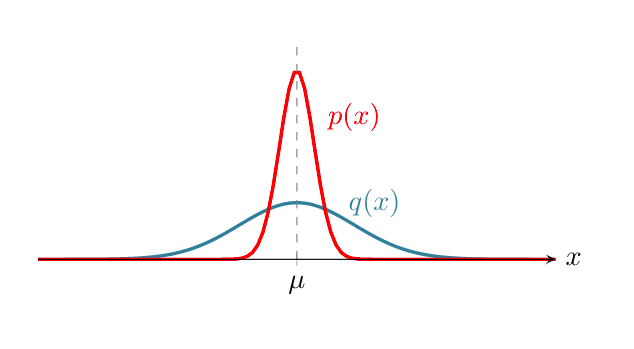#### Definition:

The variance of a random variableis represented by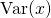and is defined by the expected value of the squared deviation from the mean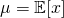: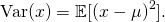is also often represented by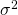as the variance is the square of the standard deviation denoted by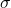.

The variance can also be expanded as: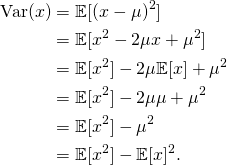Variance is closely related to covariance that represents the linear dependency between two variables. In fact, it is easy to see that: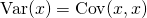#### Properties:

Sign: Variance is always non-negative: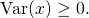Shift invariance: Variance is invariant to shift: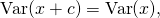where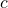is a constant.

Scaling: Scaling a random variable by, scales the variance by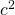:

(1)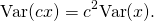Sum: The variance of sum/minus is: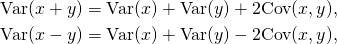where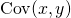denotes the covariance between two random variables. This can be easily generalized to the sum of many random variables: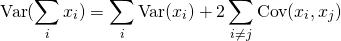If all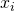are independent or uncorrelated, we have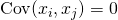,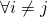. This results in:

(2)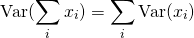#### Example:

Assumeis a Normally-distributed random variable with mean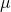and variance, i.e.,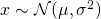. We use a Monte Carlo estimate of the mean by drawing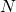samples from the distribution denoted by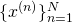: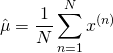what are the mean and variance of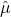?

First, we should note thatitself is a random variable. In other words, it has its own distribution with its own mean and variance. Second,is a stochastic estimation of. i.e.,is fixed in our problem, butdepends on the set of samples. If we calculateusing a different set of samples, its value will be different.

Mean: Let’s use what we learned in the previous post to derive the mean of: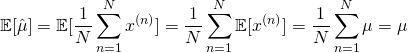So, the expected value ofis.

Variance: Since the set of samples are drawn independently, we can use the sum and scaling properties (Eq.1 and 2):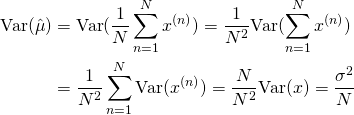As you can see, the variance ofdepends on the sample size. As the sample size grows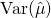goes to zero. In fact the sum of Normally-distributed random variables has a Normal distribution itself. Thus, we have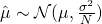. Asincreases, the distribution ofwhich is centered at, becomes narrower, and as a result,approaches.

#### Why variance is important in training:

Recall that in the previous post I mentioned that many training objective functions take the form of an expectation. The gradient of those objective functions is also in the form of an expectation. Since in general, we don’t have an analytic expression for the expected value of the gradient, we typically use a stochastic estimation of the gradient for optimizing the objective function. In this case, the variance of the gradient estimator plays a crucial role in training. High gradient variance can extremely slow down the training progress.

In practice, many factors impact the variance of a gradient estimator. Here, we are going to analyze the effect of the training batch size. Let’s consider a model with a single parameter. We represent this model by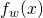whereis an input instance and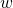is the parameter. For example, our model can be as simple as a linear function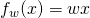. Let’s denote the target variable by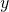and the loss function measuring the mismatch between the model outputand targetby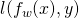. The training objective is to findthat has the lowest loss in average. This is formulated as minimizing the following expectation: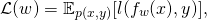where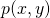is the joint distribution of input and target variables. We can use a gradient-based optimization method to minimize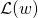. For this, we require computing the gradient of the loss function: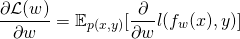As you can see both the objective functionand the gradient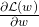are in the form of an expectation.

We don’t have the jointexplicitly. Thus, we cannot compute the expectations analytically. However, the training dataset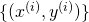are samples drawn from the joint. We can define a Monte Carlo estimate of the gradient using a mini-batch of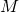randomly-selected training datapoints: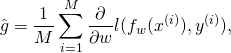where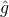is the stochastic estimator of. As we saw in the example,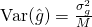where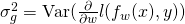. We generally don’t have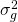, so we cannot quantify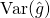. However, it is easy to see thatreduces as the mini-batch size increases. In other words, increasing the training mini-batch size, in average, bringscloser to the true gradient.

#### Summary

In this post, we learned about variance and its basic properties, and we saw how we can use them to derive the mean and variance of an estimator. In the next post, we will dig deeper into an ML problem to better understand how these fundamental concepts play together during training. If you like to stay updated with the future posts, you can use the form below to subscribe.